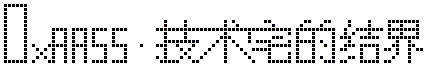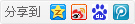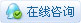##帐号 自动登录 找回密码 密码 立即注册→加入我们
 搜索 热搜: 下载 VB C 实现 编写

# 【VFB】词法约定（VFB教程2-2）

50主题 635积分

UID
3260

7

12 点

497 个

1 次

0 份

22 小时

2017-12-26发表于 2018-2-26 21:00:53 | 显示全部楼层 |阅读模式

### 欢迎访问技术宅的结界，请注册或者登录吧。

x

[Visual Basic] 纯文本查看 复制代码
' comment text

[Visual Basic] 纯文本查看 复制代码
Rem comment

[Visual Basic] 纯文本查看 复制代码
/' Multi-line
comment '/
Print "Hello" /' embedded comment'/ " world"

[Visual Basic] 纯文本查看 复制代码
Print _ ' line
"This is part of the previous line's statement"

FreeBASIC符号的命名约定

%Integer
&Long
!Single
#Double
\$String

[Visual Basic] 纯文本查看 复制代码
Dim x As Integer = 123456
Dim b As Byte = -128

“&H”，后跟十六进制数字（0 1 2 3 4 5 6 7 8 9 A B C D E F）。
[Visual Basic] 纯文本查看 复制代码
Dim x As Integer = &h1E240
Dim b As Byte = &H80

“&O”，后跟八进制数字（0 1 2 3 4 5 6 7）
[Visual Basic] 纯文本查看 复制代码
Dim x As Integer = &O361100
Dim b As Byte = &O200

“&B”，后跟二进制数字（0 1）
[Visual Basic] 纯文本查看 复制代码
Dim x As Integer = &B11110001001000000
Dim b As Byte = &B10000000

“%”被认为是32/64（取决于平台）位整数。(Integer)
“L”，“&”被视为有符号的32位长整数。(Long)
“U”被视为无符号32/64（取决于平台）位整数。(UInteger)
“UL”被视为无符号32位整数。(Ulong)
“LL”被视为带符号的64位整数。(LongInt)
“ULL”被视为无符号的64位整数。(ULongInt)

[Visual Basic] 纯文本查看 复制代码
Dim a As Long = 123L
Dim b As UInteger = &h1234u
Dim c As LongInt = 76543LL
Dim d As ULongInt = &b1010101ULL

number[.[fraction]][((D|E) [+|-] exponent)|(D|E)|][suffix]

.fraction[((D|E) [+|-] exponent)|(D|E)|][suffix]

[Visual Basic] 纯文本查看 复制代码
Dim a As Double = 123.456
Dim b As Double = -123.0

[Visual Basic] 纯文本查看 复制代码
Dim a As Double = -123.0d
Dim b As Double = -123e
Dim c As Double = 743.1e+13
Dim d As Double = 743.1D-13
Dim e As Double = 743.1E13
Dim f As Single = 743D!

[Visual Basic] 纯文本查看 复制代码
Dim a As Single = 3.1!
Dim b As Single = -123.456e-7f
Dim c As Double = 0#
Dim d As Double = 3.141592653589e3#

[Visual Basic] 纯文本查看 复制代码
Print "Hello World!"
Print "That's right!"
Print "See the ""word"" contained in double quotes."

[Visual Basic] 纯文本查看 复制代码
Print !"Hello\nWorld!"

symbolname:

symbolname 标签必须以冒号（:)字符结尾。
[Visual Basic] 纯文本查看 复制代码
Goto beginning
beginning:
Print "你好，世界！"

[Visual Basic] 纯文本查看 复制代码
Dim myvariable _
As Integer

[Visual Basic] 纯文本查看 复制代码
Declare Sub drawRectangle( ByVal x As Integer, ByVal y As Integer, _
ByVal w As Integer, ByVal h As Integer )

'' or:

Declare Sub drawRectangle _
( _
ByVal x As Integer, _
ByVal y As Integer, _
ByVal w As Integer, _
ByVal h As Integer _
)

_行连续字符可以插入一行代码中的任何一点。它不会在评论内。

[Visual Basic] 纯文本查看 复制代码
Dim As Integer a_     '错误写法
Dim As Integer a _   '正确写法
= 5本版积分规则 回帖并转播 回帖后跳转到最后一页|申请友链||Archiver|手机版|小黑屋|技术宅的结界 ( 滇ICP备16008837号 )|网站地图

GMT+8, 2020-2-22 19:36 , Processed in 0.109452 second(s), 32 queries , Gzip On.